Скачать презентацию House Blueprint Living Room 10 x 14 x 12

bb4821106a4ff8cb32113cdd3e41b58f.ppt

• Количество слайдов: 10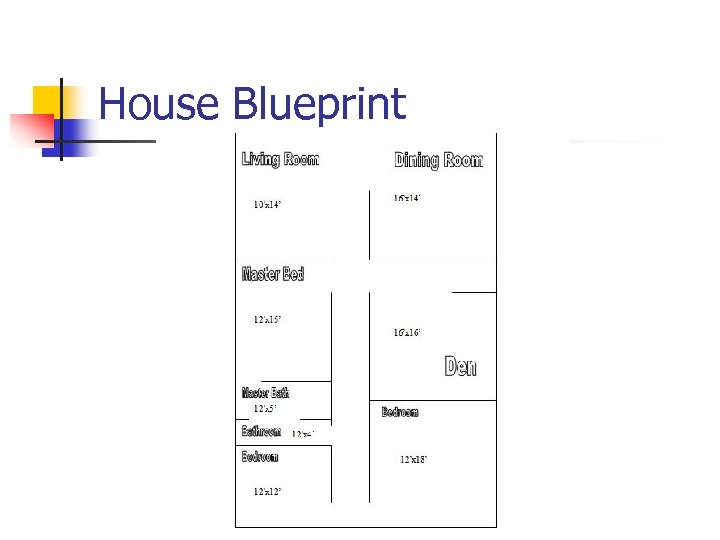House Blueprint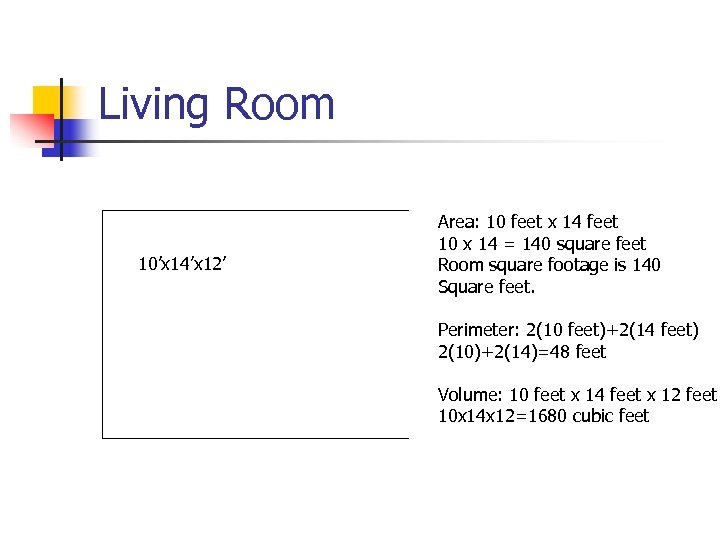Living Room 10’x 14’x 12’ Area: 10 feet x 14 feet 10 x 14 = 140 square feet Room square footage is 140 Square feet. Perimeter: 2(10 feet)+2(14 feet) 2(10)+2(14)=48 feet Volume: 10 feet x 14 feet x 12 feet 10 x 14 x 12=1680 cubic feet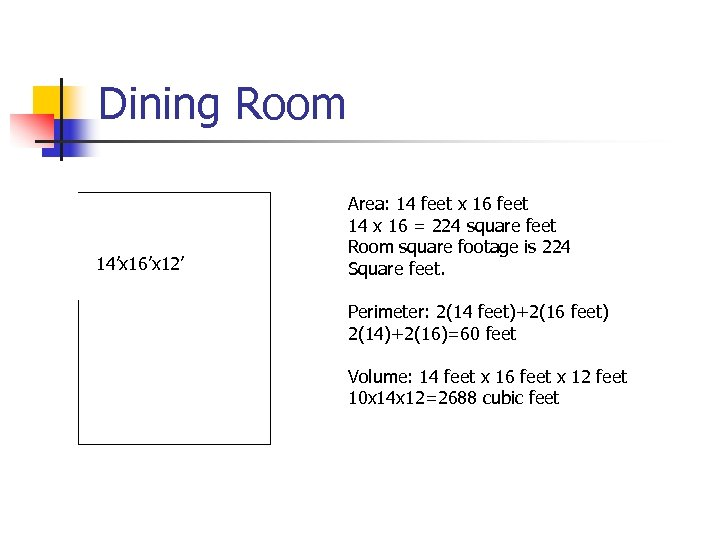Dining Room 14’x 16’x 12’ Area: 14 feet x 16 feet 14 x 16 = 224 square feet Room square footage is 224 Square feet. Perimeter: 2(14 feet)+2(16 feet) 2(14)+2(16)=60 feet Volume: 14 feet x 16 feet x 12 feet 10 x 14 x 12=2688 cubic feet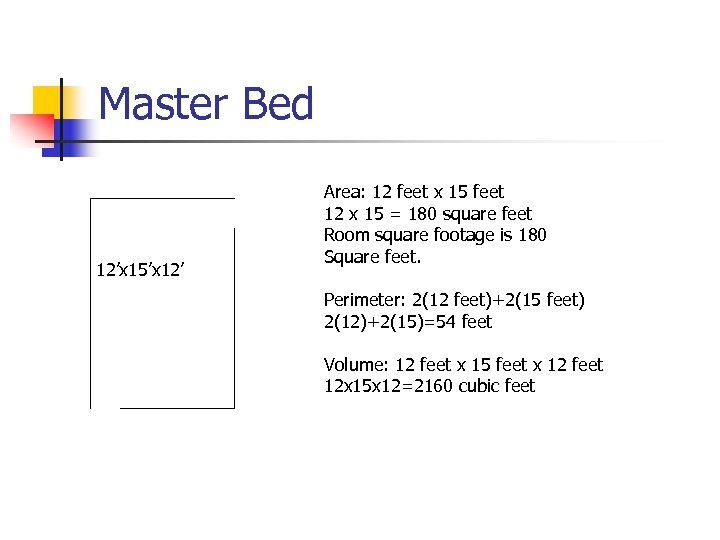Master Bed 12’x 15’x 12’ Area: 12 feet x 15 feet 12 x 15 = 180 square feet Room square footage is 180 Square feet. Perimeter: 2(12 feet)+2(15 feet) 2(12)+2(15)=54 feet Volume: 12 feet x 15 feet x 12 feet 12 x 15 x 12=2160 cubic feet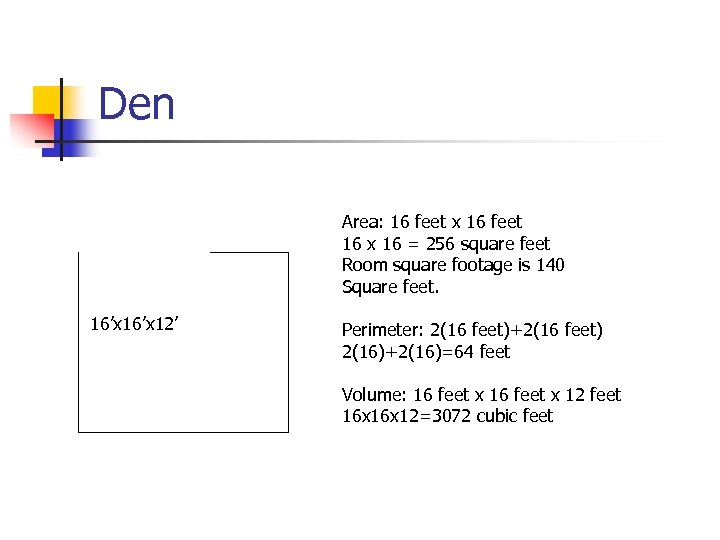Den Area: 16 feet x 16 feet 16 x 16 = 256 square feet Room square footage is 140 Square feet. 16’x 12’ Perimeter: 2(16 feet)+2(16 feet) 2(16)+2(16)=64 feet Volume: 16 feet x 12 feet 16 x 12=3072 cubic feet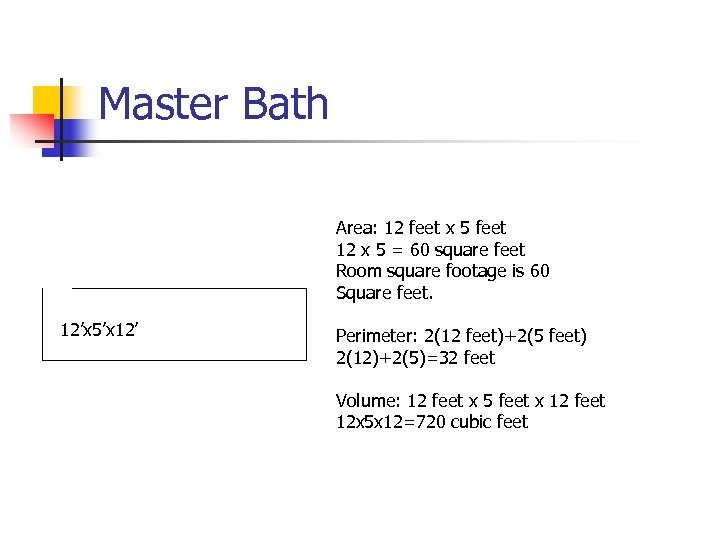Master Bath Area: 12 feet x 5 feet 12 x 5 = 60 square feet Room square footage is 60 Square feet. 12’x 5’x 12’ Perimeter: 2(12 feet)+2(5 feet) 2(12)+2(5)=32 feet Volume: 12 feet x 5 feet x 12 feet 12 x 5 x 12=720 cubic feet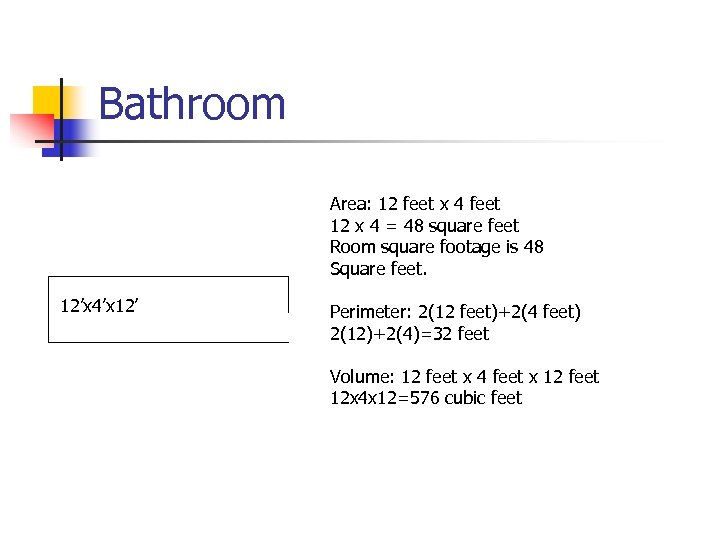Bathroom Area: 12 feet x 4 feet 12 x 4 = 48 square feet Room square footage is 48 Square feet. 12’x 4’x 12’ Perimeter: 2(12 feet)+2(4 feet) 2(12)+2(4)=32 feet Volume: 12 feet x 4 feet x 12 feet 12 x 4 x 12=576 cubic feet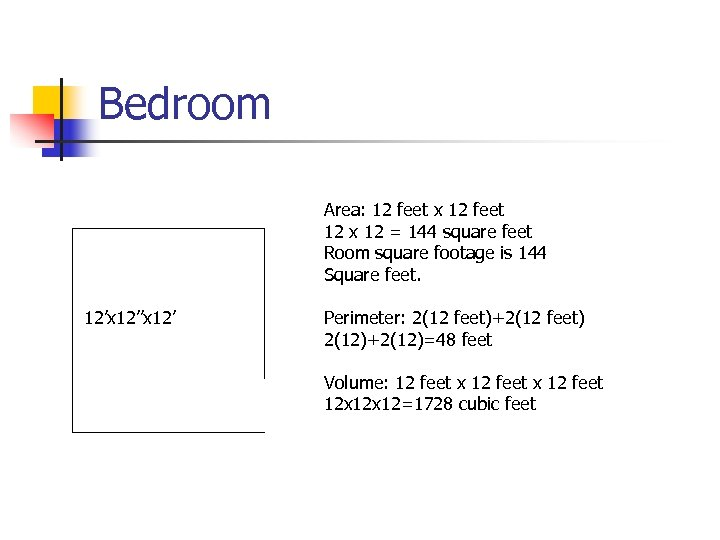Bedroom Area: 12 feet x 12 feet 12 x 12 = 144 square feet Room square footage is 144 Square feet. 12’x 12’ Perimeter: 2(12 feet)+2(12 feet) 2(12)+2(12)=48 feet Volume: 12 feet x 12 feet 12 x 12=1728 cubic feet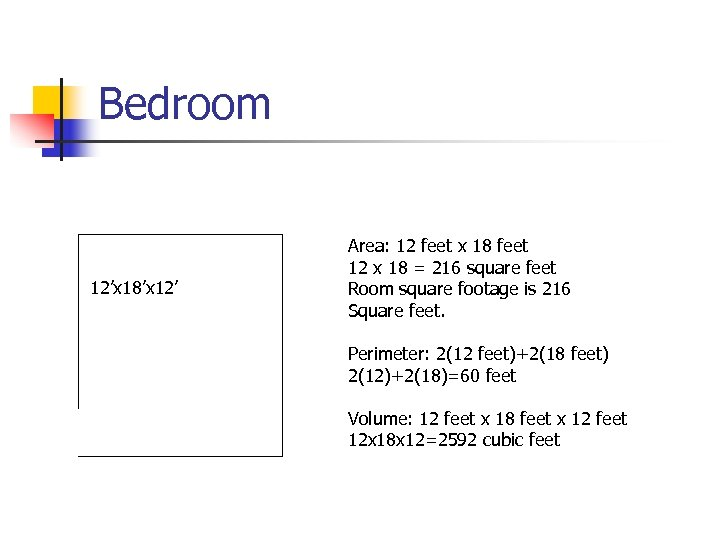Bedroom 12’x 18’x 12’ Area: 12 feet x 18 feet 12 x 18 = 216 square feet Room square footage is 216 Square feet. Perimeter: 2(12 feet)+2(18 feet) 2(12)+2(18)=60 feet Volume: 12 feet x 18 feet x 12 feet 12 x 18 x 12=2592 cubic feet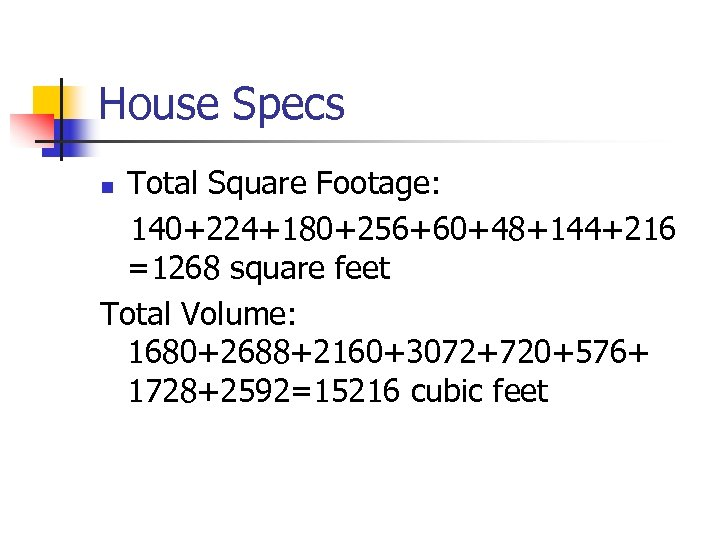House Specs Total Square Footage: 140+224+180+256+60+48+144+216 =1268 square feet Total Volume: 1680+2688+2160+3072+720+576+ 1728+2592=15216 cubic feet n